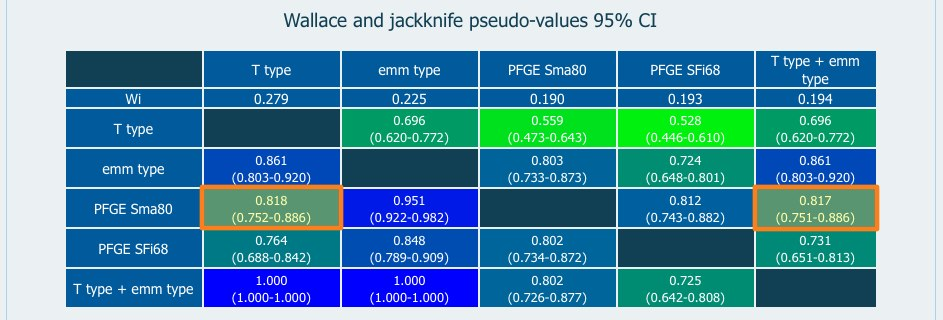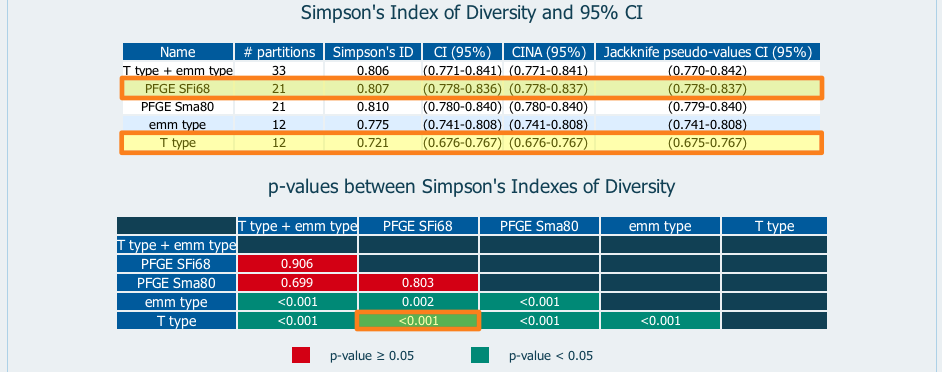Comparing Partitions
Getting started
Diversity
Pairwise agreement
Information theory
Calculations

## p-values between coefficients

Our main goal involves presenting evidence of whether there is a difference between the calculated coefficient values. For example, if two 95% Wallace's confidence intervals overlap, can they still be considered statistically different?

To reject the hypothesis that there is no difference between coefficients, we need to perform a significance test. The Comparing Partitions user has now access to a p-value, helping to decide if the null hypothesis should or should not be rejected. The p-value is the probability of wrongly rejecting the null hypothesis if it is in fact true.

H0: There is no difference between the coefficients;

H1: There is a difference between the coefficients.

One rejects the null hypothesis if the p-value is smaller than or equal to the significance level. By convention, if p-value≤0.05 one can reject the null hypothesis. This means that except for a (perhaps important) 5% chance of being wrong the researcher can reasonably reject the hypothesis that the two results are the same.

The p-value herein presented is calculated according to the jackknife pseudo-values resampling method.

### Example 1 - Wallace coefficient

• We want to test if the highlighted values of Wallace coefficients are statistically different (microbial typing test data):After the resampling procedure choose in the drop-boxes:After clicking "p-value" a new table is displayed:The resulting p-value is higher that 0.05. Therefore the null hypothesis

H0: WPFGE Sma80 → T type = WPFGE Sma80 → T type + emm type

cannot be rejected.

### Example 2 - Simpson's Index of Diversity

• We want to test if the Simpson's Index of Diversity values for PFGE SFi68 and T type (highlighted) are statistically different (microbial typing test data).The p-value for the comparison of the highlighted methods is <0.01. Because this value is lower than 0.05, the null hypothesis

H0: Simpson's IDPFGE SFi68 = Simpson's IDT type

can be rejected, meaning that the values are unlikely to be equal.

### Previous version

In a previous version of this website, the user was able to calculate confidence intervals and p-values according to the bootstrap resampling method. We recently evaluated different jackknife and bootstrap methods for calculation of CIs for pairwise agreement measures. our results shown that the jackknife pseudo-values is the most suited resampling method (Severiano et al, 2011) and therefore should be used. Here you can find a previous version of this tool where you can calculate the 95% CI and the p-values by bootstrap.